# data-science-ipython-notebooks 0,2,9,10,14,0,12,0

Recently updated with 50 new notebooks! Data science Python notebooks: Deep learning (TensorFlow, Theano, Caffe, Keras), scikit-learn, Kaggle, big data (Spark, Hadoop MapReduce, HDFS), matplotlib, pandas, NumPy, SciPy, Python essentials, AWS, and various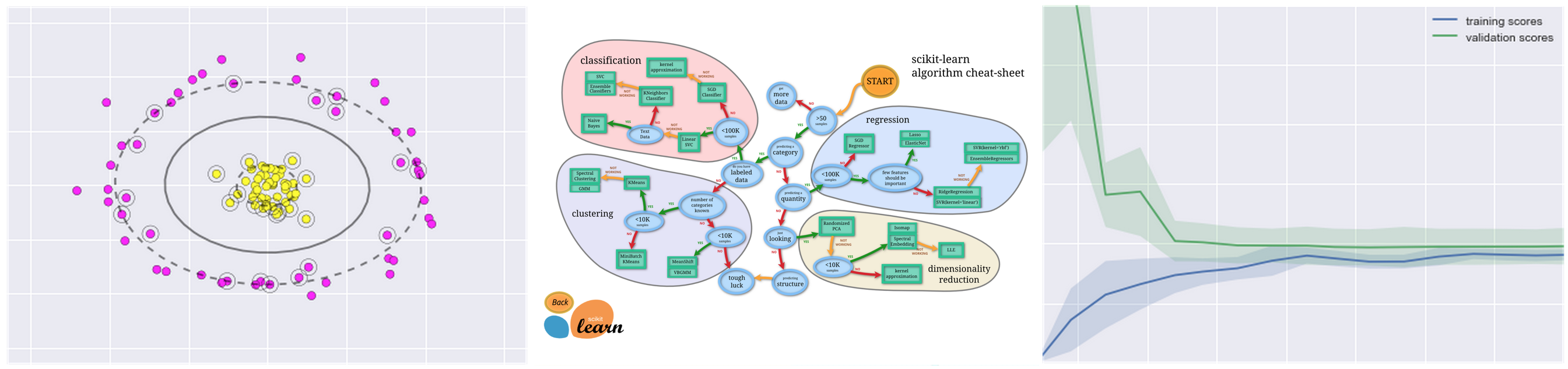# data-science-ipython-notebooks

## Index## deep-learning

IPython Notebook(s) demonstrating deep learning functionality.### tensor-flow-tutorials

Notebook Description
tsf-basics Learn basic operations in TensorFlow, a library for various kinds of perceptual and language understanding tasks from Google.
tsf-linear Implement linear regression in TensorFlow.
tsf-logistic Implement logistic regression in TensorFlow.
tsf-nn Implement nearest neighboars in TensorFlow.
tsf-alex Implement AlexNet in TensorFlow.
tsf-cnn Implement convolutional neural networks in TensorFlow.
tsf-mlp Implement multilayer perceptrons in TensorFlow.
tsf-rnn Implement recurrent neural networks in TensorFlow.
tsf-gpu Learn about basic multi-GPU computation in TensorFlow.
tsf-gviz Learn about graph visualization in TensorFlow.
tsf-lviz Learn about loss visualization in TensorFlow.

### tensor-flow-exercises

Notebook Description
tsf-not-mnist Learn simple data curation by creating a pickle with formatted datasets for training, development and testing in TensorFlow.
tsf-fully-connected Progressively train deeper and more accurate models using logistic regression and neural networks in TensorFlow.
tsf-regularization Explore regularization techniques by training fully connected networks to classify notMNIST characters in TensorFlow.
tsf-convolutions Create convolutional neural networks in TensorFlow.
tsf-word2vec Train a skip-gram model over Text8 data in TensorFlow.
tsf-lstm Train a LSTM character model over Text8 data in TensorFlow.### theano-tutorials

Notebook Description
theano-intro Intro to Theano, which allows you to define, optimize, and evaluate mathematical expressions involving multi-dimensional arrays efficiently. It can use GPUs and perform efficient symbolic differentiation.
theano-scan Learn scans, a mechanism to perform loops in a Theano graph.
theano-logistic Implement logistic regression in Theano.
theano-rnn Implement recurrent neural networks in Theano.
theano-mlp Implement multilayer perceptrons in Theano.### keras-tutorials

Notebook Description
keras Keras is an open source neural network library written in Python. It is capable of running on top of either Tensorflow or Theano.
setup Learn about the tutorial goals and how to set up your Keras environment.
intro-deep-learning-ann Get an intro to deep learning with Keras and Artificial Neural Networks (ANN).
keras-otto Learn about Keras by looking at the Kaggle Otto challenge.
ann-mnist%20A%20Simple%20Implementation%20of%20ANN%20for%20MNIST.ipynb) Review a simple implementation of ANN for MNIST using Keras.
conv-nets Learn about Convolutional Neural Networks (CNNs) with Keras.
conv-net-1 Recognize handwritten digits from MNIST using Keras - Part 1.
conv-net-2 Recognize handwritten digits from MNIST using Keras - Part 2.
keras-models Use pre-trained models such as VGG16, VGG19, ResNet50, and Inception v3 with Keras.
auto-encoders Learn about Autoencoders with Keras.
rnn-lstm Learn about Recurrent Neural Networks (RNNs) with Keras.
lstm-sentence-gen%20LSTM%20for%20Sentence%20Generation.ipynb) Learn about RNNs using Long Short Term Memory (LSTM) networks with Keras.

### deep-learning-misc

Notebook Description
deep-dream Caffe-based computer vision program which uses a convolutional neural network to find and enhance patterns in images.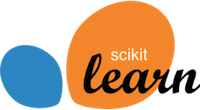## scikit-learn

IPython Notebook(s) demonstrating scikit-learn functionality.

Notebook Description
intro Intro notebook to scikit-learn. Scikit-learn adds Python support for large, multi-dimensional arrays and matrices, along with a large library of high-level mathematical functions to operate on these arrays.
knn Implement k-nearest neighbors in scikit-learn.
linear-reg Implement linear regression in scikit-learn.
svm Implement support vector machine classifiers with and without kernels in scikit-learn.
random-forest Implement random forest classifiers and regressors in scikit-learn.
k-means Implement k-means clustering in scikit-learn.
pca Implement principal component analysis in scikit-learn.
gmm Implement Gaussian mixture models in scikit-learn.
validation Implement validation and model selection in scikit-learn.## statistical-inference-scipy

IPython Notebook(s) demonstrating statistical inference with SciPy functionality.

Notebook Description
scipy SciPy is a collection of mathematical algorithms and convenience functions built on the Numpy extension of Python. It adds significant power to the interactive Python session by providing the user with high-level commands and classes for manipulating and visualizing data.
effect-size Explore statistics that quantify effect size by analyzing the difference in height between men and women. Uses data from the Behavioral Risk Factor Surveillance System (BRFSS) to estimate the mean and standard deviation of height for adult women and men in the United States.
sampling Explore random sampling by analyzing the average weight of men and women in the United States using BRFSS data.
hypothesis Explore hypothesis testing by analyzing the difference of first-born babies compared with others.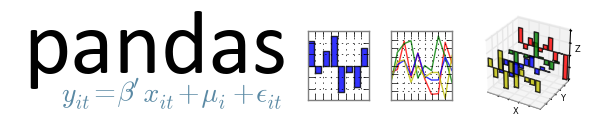## pandas

IPython Notebook(s) demonstrating pandas functionality.

Notebook Description
pandas Software library written for data manipulation and analysis in Python. Offers data structures and operations for manipulating numerical tables and time series.
github-data-wrangling Learn how to load, clean, merge, and feature engineer by analyzing GitHub data from the `Viz` repo.
Introduction-to-Pandas Introduction to Pandas.
Data Indexing and Selection Learn about data indexing and selection in Pandas.
Operations-in-Pandas Learn about operating on data in Pandas.
Missing-Values Learn about handling missing data in Pandas.
Hierarchical-Indexing Learn about hierarchical indexing in Pandas.
Concat-And-Append Learn about combining datasets: concat and append in Pandas.
Merge-and-Join Learn about combining datasets: merge and join in Pandas.
Aggregation-and-Grouping Learn about aggregation and grouping in Pandas.
Pivot-Tables Learn about pivot tables in Pandas.
Working-With-Strings Learn about vectorized string operations in Pandas.
Working-with-Time-Series Learn about working with time series in pandas.
Performance-Eval-and-Query Learn about high-performance Pandas: eval() and query() in Pandas.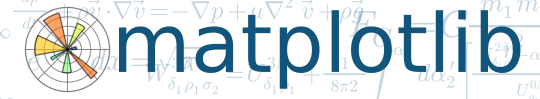## matplotlib

IPython Notebook(s) demonstrating matplotlib functionality.

Notebook Description
matplotlib Python 2D plotting library which produces publication quality figures in a variety of hardcopy formats and interactive environments across platforms.
matplotlib-applied Apply matplotlib visualizations to Kaggle competitions for exploratory data analysis. Learn how to create bar plots, histograms, subplot2grid, normalized plots, scatter plots, subplots, and kernel density estimation plots.
Introduction-To-Matplotlib Introduction to Matplotlib.
Simple-Line-Plots Learn about simple line plots in Matplotlib.
Simple-Scatter-Plots Learn about simple scatter plots in Matplotlib.
Errorbars.ipynb Learn about visualizing errors in Matplotlib.
Density-and-Contour-Plots Learn about density and contour plots in Matplotlib.
Histograms-and-Binnings Learn about histograms, binnings, and density in Matplotlib.
Customizing-Legends Learn about customizing plot legends in Matplotlib.
Customizing-Colorbars Learn about customizing colorbars in Matplotlib.
Multiple-Subplots Learn about multiple subplots in Matplotlib.
Text-and-Annotation Learn about text and annotation in Matplotlib.
Customizing-Ticks Learn about customizing ticks in Matplotlib.
Settings-and-Stylesheets Learn about customizing Matplotlib: configurations and stylesheets.
Three-Dimensional-Plotting Learn about three-dimensional plotting in Matplotlib.
Geographic-Data-With-Basemap Learn about geographic data with basemap in Matplotlib.
Visualization-With-Seaborn Learn about visualization with Seaborn.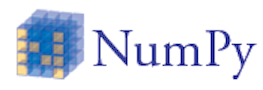## numpy

IPython Notebook(s) demonstrating NumPy functionality.

Notebook Description
numpy Adds Python support for large, multi-dimensional arrays and matrices, along with a large library of high-level mathematical functions to operate on these arrays.
Introduction-to-NumPy Introduction to NumPy.
Understanding-Data-Types Learn about data types in Python.
The-Basics-Of-NumPy-Arrays Learn about the basics of NumPy arrays.
Computation-on-arrays-ufuncs Learn about computations on NumPy arrays: universal functions.
Computation-on-arrays-aggregates Learn about aggregations: min, max, and everything in between in NumPy.
Fancy-Indexing Learn about fancy indexing in NumPy.
Sorting Learn about sorting arrays in NumPy.
Structured-Data-NumPy Learn about structured data: NumPy’s structured arrays.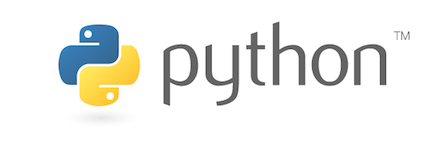## python-data

IPython Notebook(s) demonstrating Python functionality geared towards data analysis.

Notebook Description
data structures Learn Python basics with tuples, lists, dicts, sets.
data structure utilities Learn Python operations such as slice, range, xrange, bisect, sort, sorted, reversed, enumerate, zip, list comprehensions.
functions Learn about more advanced Python features: Functions as objects, lambda functions, closures, *args, **kwargs currying, generators, generator expressions, itertools.
datetime Learn how to work with Python dates and times: datetime, strftime, strptime, timedelta.
logging Learn about Python logging with RotatingFileHandler and TimedRotatingFileHandler.
pdb Learn how to debug in Python with the interactive source code debugger.
unit tests Learn how to test in Python with Nose unit tests.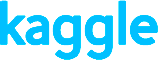IPython Notebook(s) used in kaggle competitions and business analyses.

Notebook Description
titanic Predict survival on the Titanic. Learn data cleaning, exploratory data analysis, and machine learning.
churn-analysis Predict customer churn. Exercise logistic regression, gradient boosting classifers, support vector machines, random forests, and k-nearest-neighbors. Includes discussions of confusion matrices, ROC plots, feature importances, prediction probabilities, and calibration/descrimination.## spark

IPython Notebook(s) demonstrating spark and HDFS functionality.

Notebook Description
spark In-memory cluster computing framework, up to 100 times faster for certain applications and is well suited for machine learning algorithms.
hdfs Reliably stores very large files across machines in a large cluster.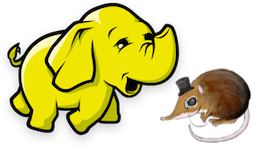## mapreduce-python

IPython Notebook(s) demonstrating Hadoop MapReduce with mrjob functionality.

Notebook Description
mapreduce-python Runs MapReduce jobs in Python, executing jobs locally or on Hadoop clusters. Demonstrates Hadoop Streaming in Python code with unit test and mrjob config file to analyze Amazon S3 bucket logs on Elastic MapReduce. Disco is another python-based alternative.## aws

IPython Notebook(s) demonstrating Amazon Web Services (AWS) and AWS tools functionality.

Also check out:

• SAWS: A Supercharged AWS command line interface (CLI).
• Awesome AWS: A curated list of libraries, open source repos, guides, blogs, and other resources.
Notebook Description
boto Official AWS SDK for Python.
s3cmd Interacts with S3 through the command line.
s3distcp Combines smaller files and aggregates them together by taking in a pattern and target file. S3DistCp can also be used to transfer large volumes of data from S3 to your Hadoop cluster.
s3-parallel-put Uploads multiple files to S3 in parallel.
redshift Acts as a fast data warehouse built on top of technology from massive parallel processing (MPP).
kinesis Streams data in real time with the ability to process thousands of data streams per second.
lambda Runs code in response to events, automatically managing compute resources.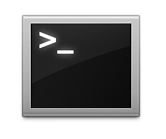## commands

IPython Notebook(s) demonstrating various command lines for Linux, Git, etc.

Notebook Description
linux Unix-like and mostly POSIX-compliant computer operating system. Disk usage, splitting files, grep, sed, curl, viewing running processes, terminal syntax highlighting, and Vim.
anaconda Distribution of the Python programming language for large-scale data processing, predictive analytics, and scientific computing, that aims to simplify package management and deployment.
ipython notebook Web-based interactive computational environment where you can combine code execution, text, mathematics, plots and rich media into a single document.
git Distributed revision control system with an emphasis on speed, data integrity, and support for distributed, non-linear workflows.
ruby Used to interact with the AWS command line and for Jekyll, a blog framework that can be hosted on GitHub Pages.
jekyll Simple, blog-aware, static site generator for personal, project, or organization sites. Renders Markdown or Textile and Liquid templates, and produces a complete, static website ready to be served by Apache HTTP Server, Nginx or another web server.
pelican Python-based alternative to Jekyll.
django High-level Python Web framework that encourages rapid development and clean, pragmatic design. It can be useful to share reports/analyses and for blogging. Lighter-weight alternatives include Pyramid, Flask, Tornado, and Bottle.

## misc

IPython Notebook(s) demonstrating miscellaneous functionality.

Notebook Description
regex Regular expression cheat sheet useful in data wrangling.
algorithmia Algorithmia is a marketplace for algorithms. This notebook showcases 4 different algorithms: Face Detection, Content Summarizer, Latent Dirichlet Allocation and Optical Character Recognition.

## notebook-installation

### anaconda

Anaconda is a free distribution of the Python programming language for large-scale data processing, predictive analytics, and scientific computing that aims to simplify package management and deployment.

Follow instructions to install Anaconda or the more lightweight miniconda.

### dev-setup

For detailed instructions, scripts, and tools to set up your development environment for data analysis, check out the dev-setup repo.

### running-notebooks

To view interactive content or to modify elements within the IPython notebooks, you must first clone or download the repository then run the notebook. More information on IPython Notebooks can be found here.

``````\$ git clone https://github.com/donnemartin/data-science-ipython-notebooks.git
\$ cd data-science-ipython-notebooks
\$ jupyter notebook
``````

Notebooks tested with Python 2.7.x.

## contributing

Contributions are welcome! For bug reports or requests please submit an issue.

## contact-info

Feel free to contact me to discuss any issues, questions, or comments.

This repository contains a variety of content; some developed by Donne Martin, and some from third-parties. The third-party content is distributed under the license provided by those parties.

I am providing code and resources in this repository to you under an open source license. Because this is my personal repository, the license you receive to my code and resources is from me and not my employer (Facebook).

``````Copyright 2015 Donne Martin

you may not use this file except in compliance with the License.
You may obtain a copy of the License at

Unless required by applicable law or agreed to in writing, software
WITHOUT WARRANTIES OR CONDITIONS OF ANY KIND, either express or implied.
See the License for the specific language governing permissions and
``````

### Related Repositories

#### data-science-ipython-notebooks

Recently updated with 50 new notebooks! Data science Python notebooks: Deep learning (TensorFlow, Theano, Caffe, Keras), scikit-learn, Kaggle, big data (Spark, Hadoop MapReduce, HDFS), matplotlib, pandas, NumPy, SciPy, Python essentials, AWS, and various ...

#### urban-data-science

Course materials, IPython notebooks, tutorials, and guides for an urban data science course. ...

#### IPython-plotly

A collection of data science IPython notebooks with Plotly graphs ...

#### data_science_fun_conference_2015_pandas_in_a_hurry

iPython notebooks and data files for the 'Pandas in a Hurry' tutorial of the 2015 San Diego Data Science Fun Conference ...

#### data-science-ipython-notebooks

Continually updated data science Python notebooks: Deep learning (TensorFlow, Theano, Caffe, Keras), scikit-learn, Kaggle, big data (Spark, Hadoop MapReduce, HDFS), matplotlib, pandas, NumPy, SciPy, Python essentials, AWS, and various command lines. ...